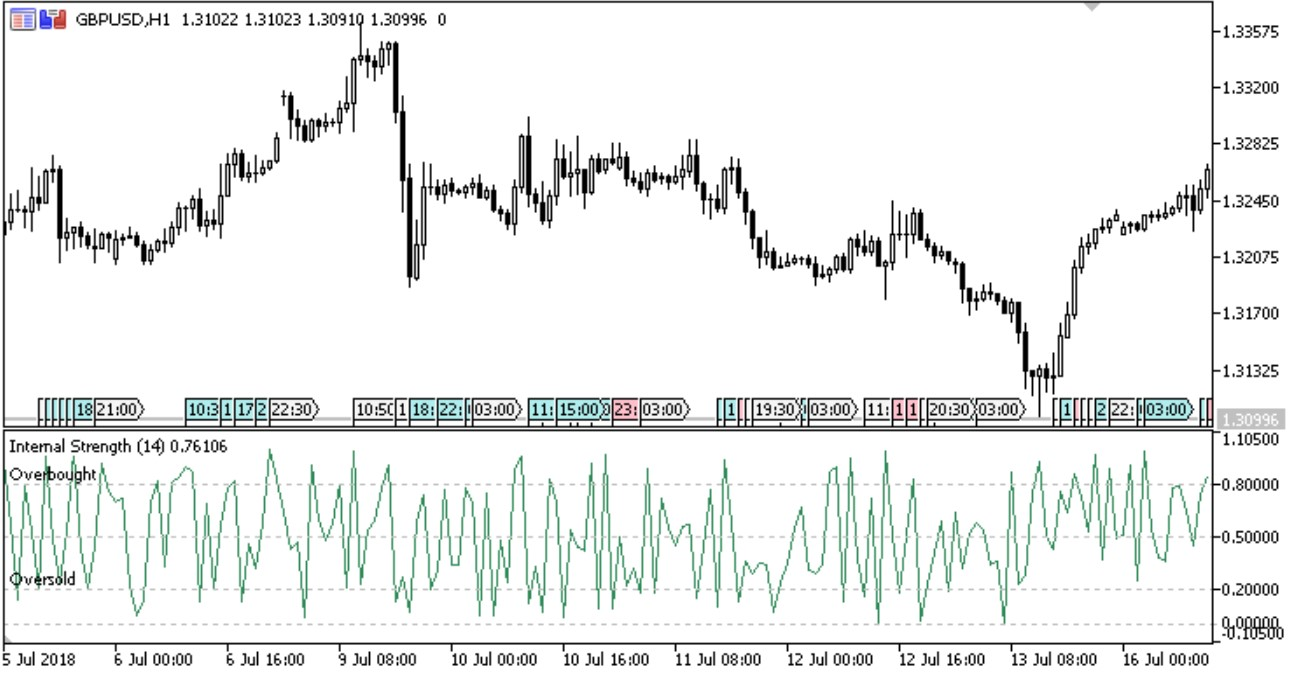# Strength oscillator Forex MT5 IndicatorThe indicator specifies the point of a daily range the price is located in. Based on the information, traders can assume further price movement and adjust their strategy accordingly.

It has four inputs:

• Calculating mode – calculation method
• HLC – by High, Low and Close prices
• RSI – RSI based on HLC
• RSI period – RSI calculation period
• Overbought – overbought level
• Oversold – oversold level

The calculation is as follows:

If Calculation mode = HLC, Internal Strength = Data
If Calculation mode = RSI, Internal Strength = RSI (Data,RSI period)4.9/5
Multibank Review4.9/5
eToro Review4.8/5
Capital.com Review

where:

Data = (Close – Low[i]) / HL HL = High – Low

Timeframe: any

Currency Pairs: any